Next: 6. Applications Up: 5.4 Implementation Details Previous: 5.4.1 Simulation Flow

## 5.4.2 Model Implementation

The simulator is designed in such a way that the simulator kernel is clearly separated from the physical models. Hence, new models can be easily implemented and added. The simulator kernel requests 4 functions of specific form which all must be provided by a new process model:

1. The first function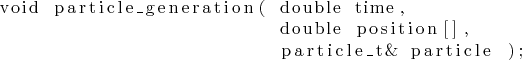generates a new particle which obeys the arriving flux distribution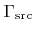. It returns a starting position and the particle properties, such as direction, energy, and species. The particle properties are stored using the data type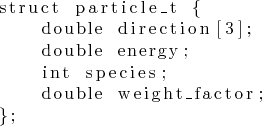which is provided by the simulator and also includes a weight factor. The initial value of the weight factor is usually 1. The starting position does not need to be set by the function, if the arriving flux distribution is spatial invariant. In this case a flag can be passed to the simulator kernel which itself chooses a random starting position on the source plane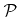.

2. The second function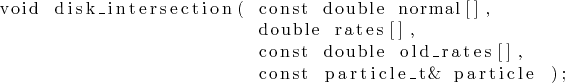is called, whenever a particle intersects the tangential disk of an active grid point. If this is the case, the function is responsible to make the corresponding contributions to the surface rates. Apart from the normal vector of the tangential disk, the old rates from the previous iteration step are provided, which are needed to solve recursive problems.

3. The third function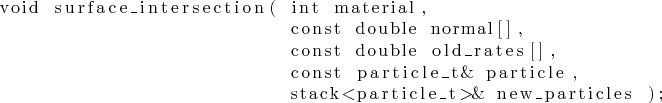describes what needs to be done if a particle intersects the surface. The current material on the surface and the surface normal (obtained by deriving the multi-linear interpolation formula within the corresponding grid cell) at the intersection point are passed to the function. Furthermore, the preceding surface rates, as needed for recursive problems, and the material type are provided. Since the rates are only stored at active grid points, the rates of the closest active grid point are taken. Together with the properties of the incident particle all information necessary in deciding whether new particles must be launched, is available. The properties of new particles, such as the reemitted direction, the energy, the particle species, and the weight factor, which all must obey the reemission probability, are simply pushed to the stack. The starting position of new particles is automatically assumed to be the surface intersection point.

4. The fourth function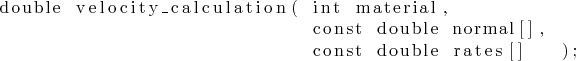is called, if the surface velocity must be known for an active grid point and for a certain material type. The normal vector is also passed to the function for the description of transport-independent surface reactions (see Section 2.3.3).

A new process model is represented as a class which contains all these functions in the public section. To simulate a certain process, the corresponding class is simply passed to the simulation algorithm. C++ offers dynamic and static polymorphism. However, especially the first three functions are called very often during the ray tracing procedure. At the same time, they usually contain only a few lines of code which is predestined for inlining. Therefore, to enable compiler optimizations, static polymorphism is applied, which is expressed in C++ by passing the class of a physical model as a template parameter to the simulator kernel.Next: 6. Applications Up: 5.4 Implementation Details Previous: 5.4.1 Simulation Flow

Otmar Ertl: Numerical Methods for Topography Simulation